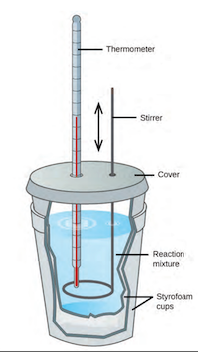Problem: If a reaction produces 1.506 kJ of heat, which is trapped in 30.0 g of water initially at 26.5°C in a calorimeter like that in Figure 5.12, what is the resulting temperature of the water?

FREE Expert Solution
89% (321 ratings)
Problem Details

If a reaction produces 1.506 kJ of heat, which is trapped in 30.0 g of water initially at 26.5°C in a calorimeter like that in Figure 5.12, what is the resulting temperature of the water?What scientific concept do you need to know in order to solve this problem?

Our tutors have indicated that to solve this problem you will need to apply the Constant-Pressure Calorimetry concept. You can view video lessons to learn Constant-Pressure Calorimetry Or if you need more Constant-Pressure Calorimetry practice, you can also practice Constant-Pressure Calorimetry practice problems .

What is the difficulty of this problem?

Our tutors rated the difficulty of If a reaction produces 1.506 kJ of heat, which is trapped in... as medium difficulty.

How long does this problem take to solve?

Our expert Chemistry tutor, Dasha took 6 minutes to solve this problem. You can follow their steps in the video explanation above.

What professor is this problem relevant for?

Based on our data, we think this problem is relevant for Professor Grant's class at HUNTER.

What textbook is this problem found in?

Our data indicates that this problem or a close variation was asked in . You can also practice practice problems .